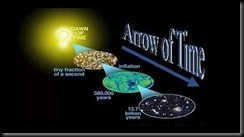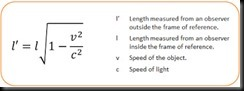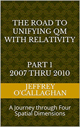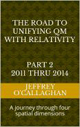The arrow of time, is the name reason given to the "one-way direction" or "asymmetry" of time by British astrophysicist Arthur Eddington in the macroscopic universe.  Its direction, according to Eddington, is determined by studying the spatial organization of atoms, molecules, and bodies, and might be drawn upon a four-dimensional relativistic map of the world.

However physical processes at the microscopic level are believed to be either entirely or mostly time-symmetric: if the direction of time were to reverse, the theoretical statements that describe them would remain true. Yet as was just mentioned at the macroscopic level it appears that this is not the case.The question as to why things appear to different on the microscopic level is an unanswered question.

Many explain the observed temporal asymmetry at the macroscopic level, the reason we see time as having a forward direction, ultimately comes down to thermodynamics, the science of heat and its relation with mechanical energy or work, and more specifically to the Second Law of Thermodynamics. That laws uses the states that the entropy of a system either remains the same or increases in every process. This phenomenon is due to the extraordinarily small probability of a decrease or that a system will return to its original configuration, based on the extraordinarily larger number of microstates in systems with greater entropy. In other Entropy  can decrease or a system can return to its original configuration, but for any macroscopic system, this outcome is so unlikely that it will never be observed in the future.

However, entropy can decrease somewhere, provided it increases somewhere else by at least as much. The entropy of a system decreases only when it interacts with some other system whose entropy increases in the process.

Yet, it is difficult to apply that definition to a quantum environment because SchrÃ¶dinger wave equation that quantum mechanics uses to determine the position component of a particle when observed does so in terms of a probability distribution over the entire universe.  Therefore, to define an arrow of time for a quantum system in terms of entropy one must show there is a physical connection between the macroscopic space-time environments we live in and a particles position in that probability field when it is observed.

Unfortunately, we define the spatial components of entropy in our macroscopic universe in terms of the space-time concepts defined by Einstein.  Therefore, to define the arrow of time in the probabilistic world associated quantum mechanics in terms of entropy we must show how it is physically connected to the spatial properties of the macroscopic universe defined by him.

Einstein gave us the ability to do this when he used the equation E=mc^2 and the constant velocity of light to define the geometric properties of space-time because it provided a method of converting a unit of time he associated with energy to unit of space.   Additionally, because the velocity of light is constant, he also defined a one to one quantitative correspondence between the both the relativistic and physical properties of a space-time universe and one made up of only four *spatial* dimensions.

Dong so allow will one to physically connect the probabilities associated with SchrÃ¶dinger’s wave equation to the Thermodynamic laws that governor the entropy in our macroscopic universe.

For example, the article â€œ Why is energy/mass quantized? â€ Oct 4, 2007 showed one can derive the quantum mechanical wave/particle properties of matter in terms of an energy wave on a "surface" of a three-dimensional space manifold with respect to fourth spatial dimension by extrapolating our understanding of a resonant structure created by a wave in a three-dimensional environment.

Briefly it showed the four conditions required for resonance to occur in a three-dimensional environment, an object, or substance with a natural frequency, a forcing function at the same frequency as the natural frequency, the lack of a damping frequency and the ability for the substance to oscillate spatial would occur in one consisting of four spatial dimensions.

The existence of four *spatial* dimensions would give an electromagnetic wave the ability to oscillate spatially on a "surface" between a third and fourth *spatial* dimensions thereby fulfilling one of the requirements for classical resonance to occur.

These oscillations would be caused by an event such as the decay of a subatomic particle or the shifting of an electron in an atomic orbital. This would force the "surface" of a three-dimensional space manifold to oscillate with the frequency associated with the energy of that event.

The oscillations caused by such an event would serve as forcing function allowing a resonant system or "structure" to be established space.

Therefore, these oscillations in a "surface" of a three-dimensional space manifold would meet the requirements mentioned above for the formation of a resonant system or "structure" in four-dimensional space if one extrapolated them to that environment.

In our three-dimensional environment the energy of a resonant system can only take on the discrete or quantized values associated with its fundamental or a harmonic of its fundamental frequency.

Hence, these resonant systems in four *spatial* dimensions would be responsible for the discrete quantized energy quantum mechanical associates with the particle properties of matter.

Yet one can also define its boundary conditions of its resonate structure in the terms of our perceptions of a three-dimensional environment.

For example, in our three-dimensional world, a point on the two-dimensional surface of paper is confined to that surface. However, that surface can oscillate up or down with respect to three-dimensional space.

Similarly, an object occupying a volume of three-dimensional space would be confined to it however, it could, similar to the surface of the paper oscillate up  or down with respect to a fourth *spatial* dimension.

It is the confinement of the upward and downward oscillations of an energy with respect to a fourth *spatial* dimension by an observation is what defines the spatial boundaries associated with a particle in the article Why is energy/mass quantized? " Oct 4, 2007.

This shows the reason Quantum Mechanics can define matter in terms of a particle/wave duality and why it only presents its particle or position properties when it is observed is because its wave component is only confined to three-dimensional space when an observation is made.

However, as mentioned earlier it also provides a way to physical connect the probabilistic environment defined by SchrÃdinger wave equation to the physicality of Einstein’s relativistic universe.

The physics of wave mechanics tell us that due to the continuous properties of the wave component associated with a quantum system it would be distributed throughout the entire "surface" a three-dimensional space manifold with respect to a fourth *spatial* dimension.

For example, the energy of a vibrating or oscillating ball on a rubber diaphragm would be disturbed over its entire surface while the magnitude of those vibrations would decrease as one move away from the focal point of the oscillations.

Similarly, if the assumption outlined above, that quantum properties of matter are a result of vibrations or oscillations in a "surface" of three-dimensional space is correct those oscillations would be distributed over the entire "surface" three-dimensional space while the magnitude of those vibrations would be greatest at the focal point of the oscillations and decreases as one moves away from it.(Some may question the fact that the energy wave associated with particle would be simultaneously distributed over the entire universe.  However, the relativistic properties of space-time tell us the distance perceived by objects or particles in relative motion is dependent on their velocity which become zero at the speed of light.  Therefore, from the perspective of an energy wave moving at the speed of light, the distance between all points in the universe along its velocity vector is zero.  In other words, because its electromagnetic wave component of a particle is moving at the speed of light as all electromagnetic0 energy must is it would be distributed or simultaneous exists at every point in the universe along its velocity vector.  There can be no other conclusion if one accepts the validity of Einstein’s theories.)

As mentioned earlier the article â€œ Why is energy/mass quantized? â€ shown a wave/particle duality of matter can be understood in terms of a resonant structure formed wave energy on the "surface" of a three-dimensional space manifold with respect to a fourth *spatial* dimension.

Yet the science of Wave Mechanics tells us resonance would most probably occur on the surface of the rubber sheet were the magnitude of the vibrations is greatest and would diminish as one move away from that point,

Similarly, a particle would most probably be observed were the magnitude of the vibrations in a "surface" of a three-dimensional space manifold is greatest and would diminish as one move away from that point.

This demonstrates that one can interconnect probabilities associated with SchrÃ¶dinger’s wave equation to the physicality of the Einstein’s Relativistic universe.

As was mentioned earlier the arrow of time is defined in classical system in terms of entropy or the level of randomness (or disorder) of a system and the Second law of thermodynamics which states that there is an the extraordinarily small probability that a system will return to its original configuration, based on the extraordinarily larger number of microstates in systems with greater entropy even though its.

Additionally, the above discussion also shows one can use the same definition for the arrow of time in a quantum universe as the one used in a macroscopic one because the position of a particle in a quantum can only be determine with respect to other particles in probability field Schrodinger’s equation.  Therefore, due to the fact that there are infinite number of possibilities in the probabilistic universe of quantum mechanics there an extraordinarily small chance of that universe retuning to is original configuration when an observation is made in the future.

Later Jeff

 The Road to Unifying QM with Relativity part 1 2007 thru 2010Ebook \$8.00 Paper Back \$15.00 The Road to Unifying QM with Relativity part 2 2011 thru 2014Ebook \$8.00 Paper Back \$16.00 The Road to Unifying QM with Relativity part 3 2015 thru 2020Ebook \$8.00 Paper Back \$18.00